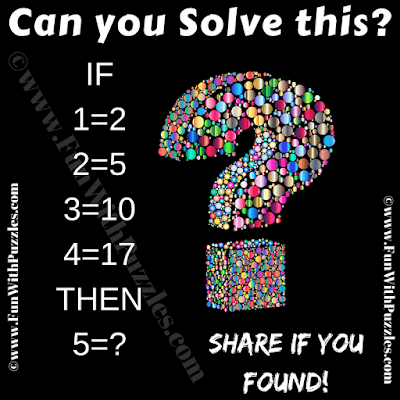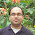## Sunday, February 17, 2019

Here is Math Logic Brain Teaser in which your challenge is find the logical pattern in the given number equations and then find the value of the missing number. Can you solve this Math Logic Puzzle without looking at the Answer?
Note that 5=2 is not correct answer. Try to decipher the hidden logic and solve this brain teaser.Can you solve this Math Logic Brain Teaser?

Answer of this "Math Logic Brain Teaser", can be viewed by clicking on answer button. Please do give your best try before looking at the answer.

#### 4 comments:

1.No. 5 = 2 per the definition given in the problem.

1.Even though, I tried to use different logic for this brainteaser, but your answer is perfectly fine.

2.1.Yes, your answer for this brainteaser match will our logic.

A comment doesn't cost a thing. Please drop a comment below to boost the author's morale.